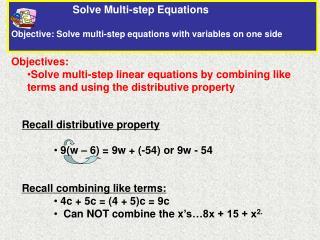DownloadDownload PresentationObjectives:

# Objectives:

Télécharger la présentation## Objectives:

- - - - - - - - - - - - - - - - - - - - - - - - - - - E N D - - - - - - - - - - - - - - - - - - - - - - - - - - -
##### Presentation Transcript

1. Solve Multi-step Equations Objective: Solve multi-step equations with variables on one side • Objectives: • Solve multi-step linear equations by combining like terms and using the distributive property • Recall distributive property • 9(w – 6) = 9w + (-54) or 9w - 54 • Recall combining like terms: • 4c + 5c = (4 + 5)c = 9c • Can NOT combine the x’s…8x + 15 + x2.

2. 3a+ 6 + a = 90 Check: 3(21)+ 6 + 21 90 Substitute 21 for a. 63+ 6 + 21 90 90 = 90 Solve a two-step Linear Equation Step 1. Combine like terms Step 2. Circle term with variable Step 3. Do all addition and subtraction to get like terms on one side Step 4. Simplify Step 5. Do multiplication and division Step 6. Check your answer Solve 3a + 6 + a = 90 4a+ 6 = 90Combine like terms. 4a + 6 – 6 = 90 – 6Subtract 6 from each side. 4a = 84Simplify. 4a4 844 = Divide each side by 4. a = 21Simplify.

3. Try It! 2x2 142 = Divide each side by 2. 3b3 843 = Divide each side by 4. b = 28Simplify. Solve a two-step Linear Equation Solve 2b + 6 + b = 90 3b + 6 = 90Combine like terms. 3b + 6 – 6 = 90 – 6Subtract 6 from each side. 3b = 84Simplify. Solve a two-step Linear Equation with Grouping Symbols Solve 2(x – 3) = 8 2x– 6 = 8 Use the Distributive Property. 2x– 6 + 6 = 8 + 6Add 6 to each side. 2x = 14 Simplify. x = 7 Simplify.

4. 2x+ 25 = 63 Combine like terms. 2x+ 25 – 25 = 63 – 25Subtract 25 from each side. 2x2 382 = Divide each side by 2. Real-world: Write and Solve a Two-step Linear Equation You need to build a rectangular pen in your back yard for your dog. One side of the pen will be against the house. Two sides of the pen have a length of x ft and the width will be 25 ft. What is the greatest length the pen can be if you have 63 ft of fencing? Draw and label a diagram to model the problem. Define a variable Write an equation x+ 25 + x = 63 2x = 38 Simplify. x = 19 The pen can be 19 ft long.

5. Solve Linear Equations with Fractions Two Methods: 1. Find common denominator 2. Multiply to clear fractions Method 1: Find a common denominator

6. Method 2: Multiply to Clear Fractions Multiply each term by 10 (common multiple of 2 and 5) Rewrite equation using distributive property Simplify Simplify Combine like terms Division Property of Equality

7. Try It! Linear Equations and Fractions Multiply each term by 8 Simplify Subtraction Property of Equality Simplify Division Property of Equality

8. Real-World: The Canoe Trip John and two friends went on a canoe trip. The cost of renting the canoe is \$5.00 per hour. In addition, each person must rent a life jacket which costs \$2.00. They spent a total of \$41.00. For how many hours did they rent the canoe? 1. Define the variable h=numbers of hours for canoe 2. Write an equation relating total cost, # hours and cost of life vests. 5.00h + 3(2.00) = 41 3. Solve the equation for h. 5.00h + 3(2.00) = 41 5.00h + 6.00 – 6.00 = 41 -6.00 5.00h = 35 h = 7

9. Summary for Solving a Multi-Step Linear Equation • Clear equations of fractions and decimals • 2. Use the distributive property to remove parentheses on each side • Combine like terms • Undo addition or subtraction • 5. Undo multiplication and division